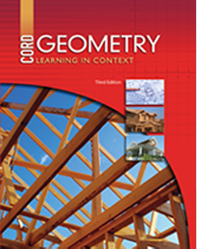# Geometry - Third Edition

## Chapter 3: Triangle Theorems

Some links are repeated for use with more than one lesson.

### 3.1 The Triangle Sum Theorem

http://www.conservapedia.com/Triangle_Angle_Sum_Theorem

http://www.mathsisfun.com/geometry/interior-angles-polygons.html
NEW This page illustrates the sum of interior angles for triangles, and several other shapes.

http://www.mathopenref.com/triangleinternalangles.html
NEW This interactive applet shows how the sum of the interior angles for any triangle always add to 180 degrees.

### 3.2 Inequalities in Geometry

http://www.regentsprep.org/regents/math/geometry/GP7/indexGP7.htm
NEW This page contains explanations and practice problems on several triangle inequality theorems.

http://www.regentsprep.org/Regents/math/geometry/GP3b/indexGP3b.htm

### 3.3 The Triangle Inequality Theorem

http://www.mathopenref.com/triangleinequality.html
This page contains an explanation of the triangle inequality theorem with a movable triangle to demonstrate.

http://www.quia.com/cm/94303.html?AP_rand=1353796656
This site has a game to practice determining if a triangle could exist based on the length of its sides.

### 3.4 Congruent Triangles

http://www.freemathhelp.com/feliz-congruent-triangles.html
This page defines congruent triangles and the qualifications for them and lists the methods for proving congruency.

http://www.regentsprep.org/Regents/math/geometry/GP3b/indexGP3b.htm

### 3.5Proving Triangles Congruent

http://www.freemathhelp.com/Lessons/Geometry_Proving_Triangles_Congruent_BB.htm
This site contains a video tutorial with notes on proving triangles congruent.

http://www.sparknotes.com/math/geometry2/congruence/section2.rhtml
This page details the different methods on how to prove triangles congruent.

### 3.6 Using Congruent Triangles

http://www.algebralab.org/practice/practice.aspx?file=Geometry_UsingCongruentTriangles.xml
This site contains an online quiz about using congruent triangles.

### 3.7 Isosceles and Right Triangle Theorems

http://www.themathpage.com/atrig/isosceles-right-triangle.htm
This page discusses the isosceles right triangle theorem and has interactive questions.

### 3.8 Altitudes, Medians, and Bisectors

http://www.bbc.co.uk/scotland/education/bitesize/higher/maths/geometry/the_straight_line8_rev.shtml